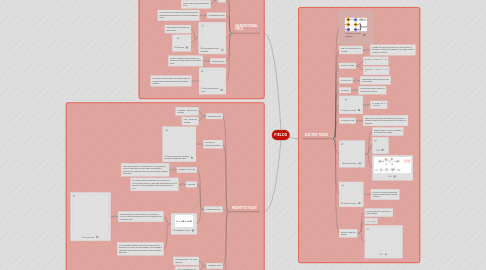# FIELDS

Get Started. It's FreeFIELDS## 1. ELECTRIC FIELDS

### 1.2. Law of Conservation of Charge

1.2.1. Charge can be transferred from one object to another, but the total charge of a closed system remains constant

### 1.3. Electric Charge

1.3.1. proton = +1.60 x 10 ^ -19 C

1.3.2. electron = -1.60 x 10 ^ -19 C

### 1.4. Conductors

1.4.1. Substances which electron can move freely

### 1.5. Insulators

1.5.1. Substances which electrons cannot move freely

### 1.6. (4) Electric Force

1.6.1. k = 8.99 x 10 ^9 N.m2/C2

### 1.7. Coulomb's Law

1.7.1. The force is inversely proportional to square of distance and directly proportional to product of charges

### 1.8. (8) Electric Field

1.8.1. Region where a force is exerted on an electric charge

1.8.2. (14)

1.8.3. (15)

### 1.9. (9) Electric Dipole

1.9.1. A pair of equal and opposite charges separated by a small distance

### 1.10. Electric Potential Energy

1.10.1. Energy stored in a system of two charges

1.10.2. 1 V = 1 J/C

1.10.3. (16)

## 2. GRAVITATIONAL FIELD

### 2.1. (1) Universal Law of Gravitation

2.1.1. Magnitude of gravitational force is directly proportional to product of masses and inversely proportional to square of distance

2.1.2. G = 6.67 x 10^-11 N.m2 / kg2

### 2.2. Mass

2.2.1. Small mass, small gravitational force

2.2.2. Large mass, large gravitational force

### 2.3. Gravitational Field

2.3.1. A collection of vectors which determine the magnitude and direction of the gravitational force

### 2.4. (6) Gravitational Field Strength

2.4.1. Magnitude of gravitational field vector

2.4.2. (2) Formula

2.5.1. Distance between the centre of a satellite and the centre of its parent body

### 2.6. (7) Geosynchronous Orbit

2.6.1. The orbit around Earth of an object with an orbital speed matching the rate of earth's rotation

## 3. MAGNETIC FIELDS

### 3.1. Magnetic poles

3.1.1. Opposite - attract one another

3.1.2. Like - repel one another

### 3.2. Principle of Electromagnetism

3.2.1. (10) Moving electric charges produce a magnetic field

### 3.3. Right-hand Rules

3.3.1. Straight Conductor

3.3.1.1. The thumb points in the direction of conventional current, while the curled fingers indicate the direction of magnetic field lines around the straight conductor.

3.3.2. Solenoid

3.3.2.1. The curled fingers indicates the direction of conventional current, while the thumb points in the direction of the magnetic fields in the center of coil.

3.3.3. (11) Magnetic Force

3.3.3.1. The right thumb in the direction of velocity or current, then the fingers point in the direction of magnetic field.

3.3.3.1.1. (12) Palm RHR

3.3.3.2. If the charge is positive, the palm indicates the direction of a force on the charge. If the charge is negative, the direction of force is in the opposite direction.

### 3.4. Magnetic Field

3.4.1. Moving parallel - no force exerted

3.4.2. Moving perpendicular - greatest force exerted

### 3.5. (5) Circular Motion

3.5.1. When initial velocity is perpendicular to the field

3.5.2. (13) Formula